# RD Sharma Solutions for Class 8 Maths Chapter 23 Data Handling - I (Classification and Tabulation of Data) Exercise 23.2

In Exercise 23.2, we shall follow an algorithm which is used to construct a grouped frequency distribution. For all these problems, RD Sharma Class 8 Solutions contain solutions which fulfil all student requirements. For further reference to the solutions of this exercise, the RD Sharma Class 8 Maths Chapter 23 is available in the pdf format, which can be accessed offline. Students can download the pdf from the links given below.

## Download the Pdf of RD Sharma Solutions for Class 8 Maths Exercise 23.2 Chapter 23 Data Handling – I (Classification and Tabulation of Data)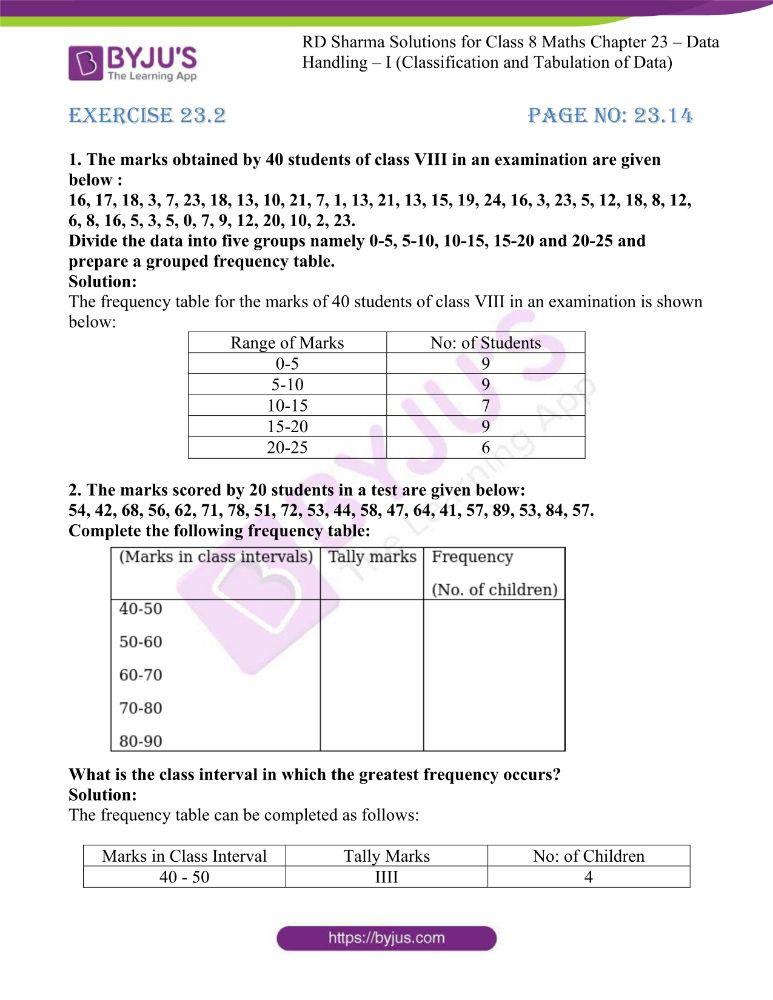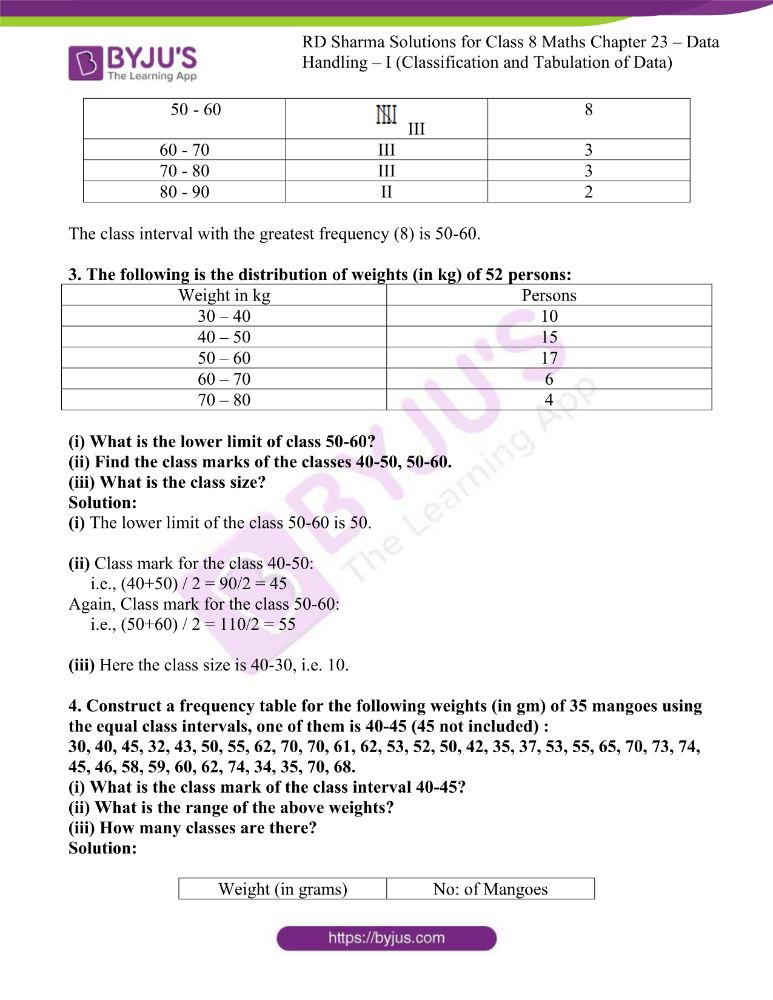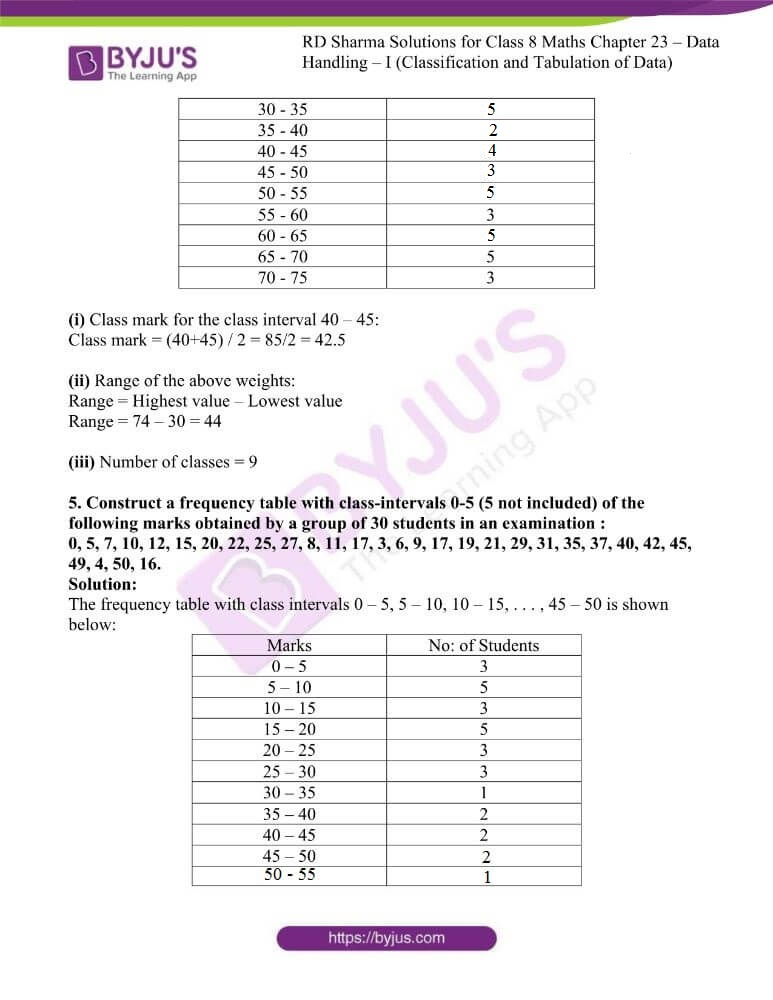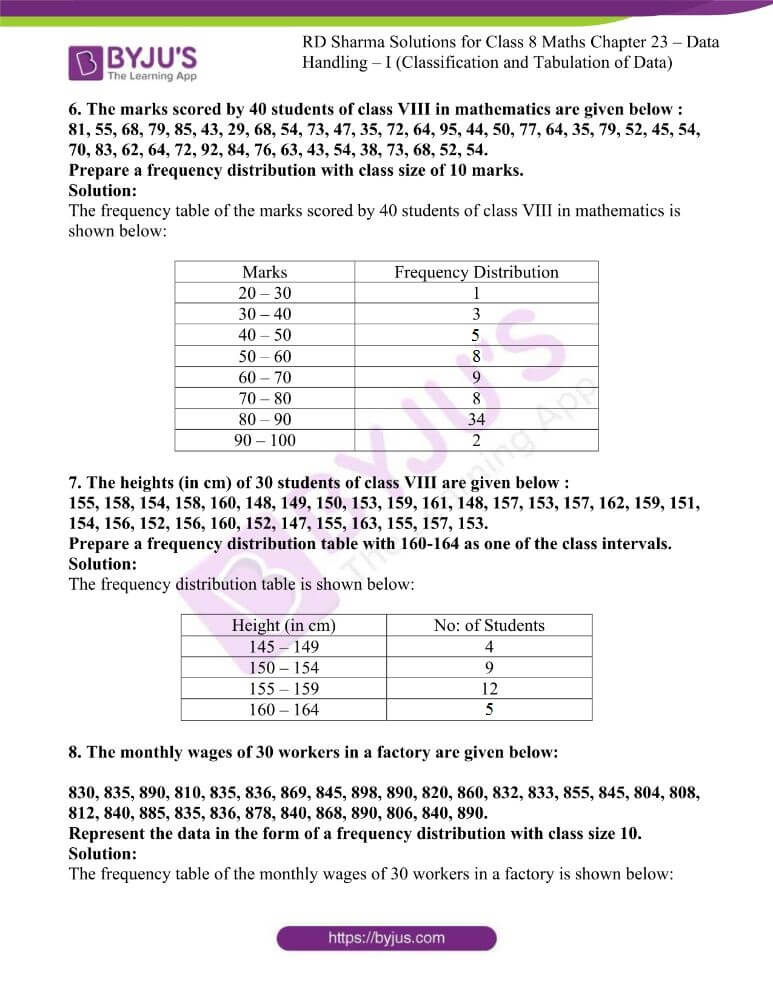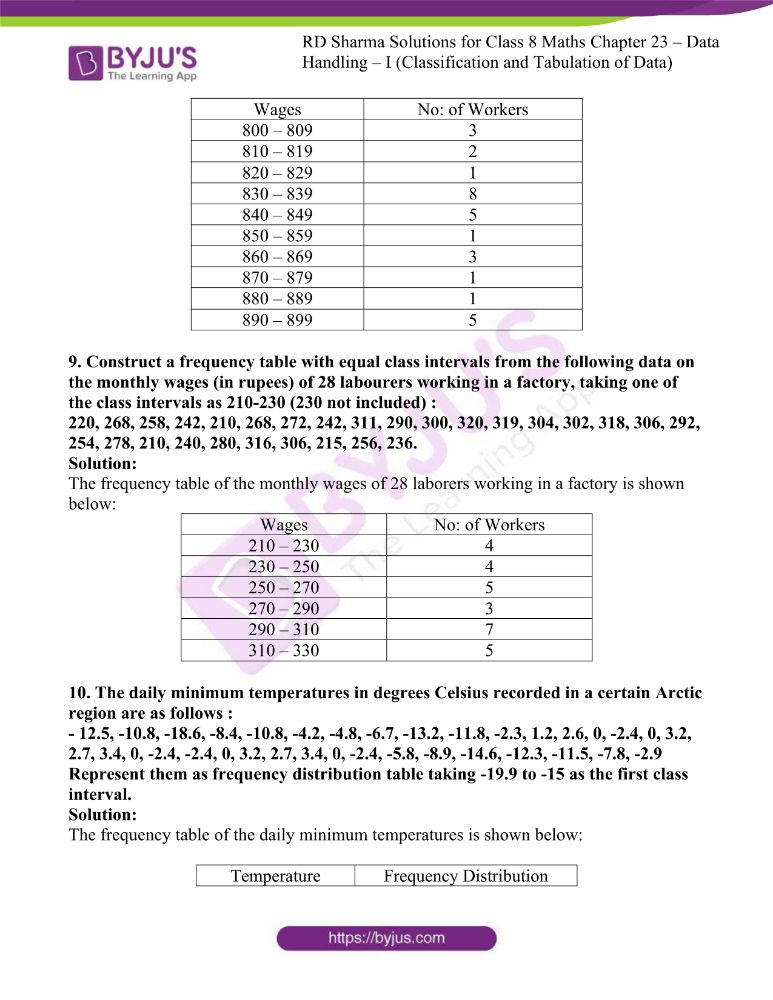### Access answers to RD Sharma Maths Solutions For Class 8 Exercise 23.2 Chapter 23 Data Handling – I (Classification and Tabulation of Data)

1. The marks obtained by 40 students of class VIII in an examination are given below :
16, 17, 18, 3, 7, 23, 18, 13, 10, 21, 7, 1, 13, 21, 13, 15, 19, 24, 16, 3, 23, 5, 12, 18, 8, 12, 6, 8, 16, 5, 3, 5, 0, 7, 9, 12, 20, 10, 2, 23.
Divide the data into five groups namely 0-5, 5-10, 10-15, 15-20 and 20-25 and prepare a grouped frequency table.

Solution:

The frequency table for the marks of 40 students of class VIII in an examination is shown below:

 Range of Marks No: of Students 0-5 9 5-10 9 10-15 7 15-20 9 20-25 6

2. The marks scored by 20 students in a test are given below:
54, 42, 68, 56, 62, 71, 78, 51, 72, 53, 44, 58, 47, 64, 41, 57, 89, 53, 84, 57.
Complete the following frequency table:What is the class interval in which the greatest frequency occurs?

Solution:

The frequency table can be completed as follows:

 Marks in Class Interval Tally Marks No: of Children 40 – 50 IIII 4 – 60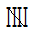III 8 60 – 70 III 3 70 – 80 III 3 80 – 90 II 2

The class interval with the greatest frequency (8) is 50-60.

3. The following is the distribution of weights (in kg) of 52 persons:

 Weight in kg Persons 30 – 40 10 40 – 50 15 50 – 60 17 60 – 70 6 70 – 80 4

(i) What is the lower limit of class 50-60?
(ii) Find the class marks of the classes 40-50, 50-60.
(iii) What is the class size?

Solution:

(i) The lower limit of the class 50-60 is 50.

(ii) Class mark for the class 40-50:

i.e., (40+50) / 2 = 90/2 = 45

Again, Class mark for the class 50-60:

i.e., (50+60) / 2 = 110/2 = 55

(iii) Here the class size is 40-30, i.e. 10.

4. Construct a frequency table for the following weights (in gm) of 35 mangoes using the equal class intervals, one of them is 40-45 (45 not included) :
30, 40, 45, 32, 43, 50, 55, 62, 70, 70, 61, 62, 53, 52, 50, 42, 35, 37, 53, 55, 65, 70, 73, 74, 45, 46, 58, 59, 60, 62, 74, 34, 35, 70, 68.
(i) What is the class mark of the class interval 40-45?
(ii) What is the range of the above weights?
(iii) How many classes are there?

Solution:

 Weight (in grams) No: of Mangoes 30 – 35 5 35 – 40 2 40 – 45 4 45 – 50 3 50 – 55 5 55 – 60 3 60 – 65 5 65 – 70 5 70 – 75 3

(i) Class mark for the class interval 40 – 45:

Class mark = (40+45) / 2 = 85/2 = 42.5

(ii) Range of the above weights:

Range = Highest value – Lowest value

Range = 74 – 30 = 44

(iii) Number of classes = 9

5. Construct a frequency table with class-intervals 0-5 (5 not included) of the following marks obtained by a group of 30 students in an examination :
0, 5, 7, 10, 12, 15, 20, 22, 25, 27, 8, 11, 17, 3, 6, 9, 17, 19, 21, 29, 31, 35, 37, 40, 42, 45, 49, 4, 50, 16.

Solution:

The frequency table with class intervals 0 – 5, 5 – 10, 10 – 15, . . . , 45 – 50 is shown below:

 Marks No: of Students 0 – 5 3 5 – 10 5 10 – 15 3 15 – 20 5 20 – 25 3 25 – 30 3 30 – 35 1 35 – 40 2 40 – 45 2 45 – 50 2 50 – 55 1

6. The marks scored by 40 students of class VIII in mathematics are given below :
81, 55, 68, 79, 85, 43, 29, 68, 54, 73, 47, 35, 72, 64, 95, 44, 50, 77, 64, 35, 79, 52, 45, 54, 70, 83, 62, 64, 72, 92, 84, 76, 63, 43, 54, 38, 73, 68, 52, 54.
Prepare a frequency distribution with class size of 10 marks.

Solution:

The frequency table of the marks scored by 40 students of class VIII in mathematics is shown below:

 Marks Frequency Distribution 20 – 30 1 30 – 40 3 40 – 50 5 50 – 60 8 60 – 70 9 70 – 80 8 80 – 90 34 90 – 100 2

7. The heights (in cm) of 30 students of class VIII are given below :
155, 158, 154, 158, 160, 148, 149, 150, 153, 159, 161, 148, 157, 153, 157, 162, 159, 151, 154, 156, 152, 156, 160, 152, 147, 155, 163, 155, 157, 153.
Prepare a frequency distribution table with 160-164 as one of the class intervals.

Solution:

The frequency distribution table is shown below:

 Height (in cm) No: of Students 145 – 149 4 150 – 154 9 155 – 159 12 160 – 164 5

8. The monthly wages of 30 workers in a factory are given below:

830, 835, 890, 810, 835, 836, 869, 845, 898, 890, 820, 860, 832, 833, 855, 845, 804, 808, 812, 840, 885, 835, 836, 878, 840, 868, 890, 806, 840, 890.
Represent the data in the form of a frequency distribution with class size 10.

Solution:

The frequency table of the monthly wages of 30 workers in a factory is shown below:

 Wages No: of Workers 800 – 809 3 810 – 819 2 820 – 829 1 830 – 839 8 840 – 849 5 850 – 859 1 860 – 869 3 870 – 879 1 880 – 889 1 890 – 899 5

9. Construct a frequency table with equal class intervals from the following data on the monthly wages (in rupees) of 28 labourers working in a factory, taking one of the class intervals as 210-230 (230 not included) :
220, 268, 258, 242, 210, 268, 272, 242, 311, 290, 300, 320, 319, 304, 302, 318, 306, 292, 254, 278, 210, 240, 280, 316, 306, 215, 256, 236.

Solution:

The frequency table of the monthly wages of 28 laborers working in a factory is shown below:

 Wages No: of Workers 210 – 230 4 230 – 250 4 250 – 270 5 270 – 290 3 290 – 310 7 310 – 330 5

10. The daily minimum temperatures in degrees Celsius recorded in a certain Arctic region are as follows :
– 12.5, -10.8, -18.6, -8.4, -10.8, -4.2, -4.8, -6.7, -13.2, -11.8, -2.3, 1.2, 2.6, 0, -2.4, 0, 3.2, 2.7, 3.4, 0, -2.4, -2.4, 0, 3.2, 2.7, 3.4, 0, -2.4, -5.8, -8.9, -14.6, -12.3, -11.5, -7.8, -2.9
Represent them as frequency distribution table taking -19.9 to -15 as the first class interval.

Solution:

The frequency table of the daily minimum temperatures is shown below:

 Temperature Frequency Distribution –19.9 to –15 1 –15 to –11.1 6 –11.1 to –6.2 6 –6.2 to –1.3 9 –1.3 to 3.6 13